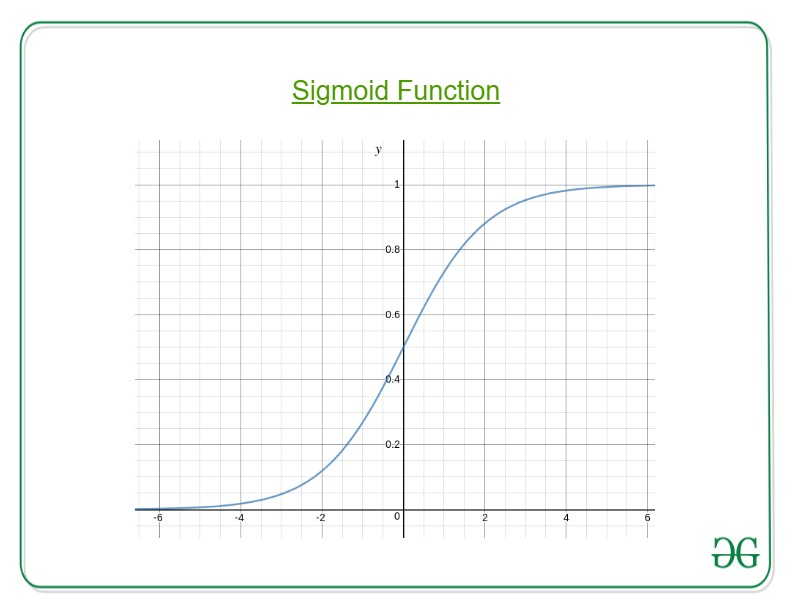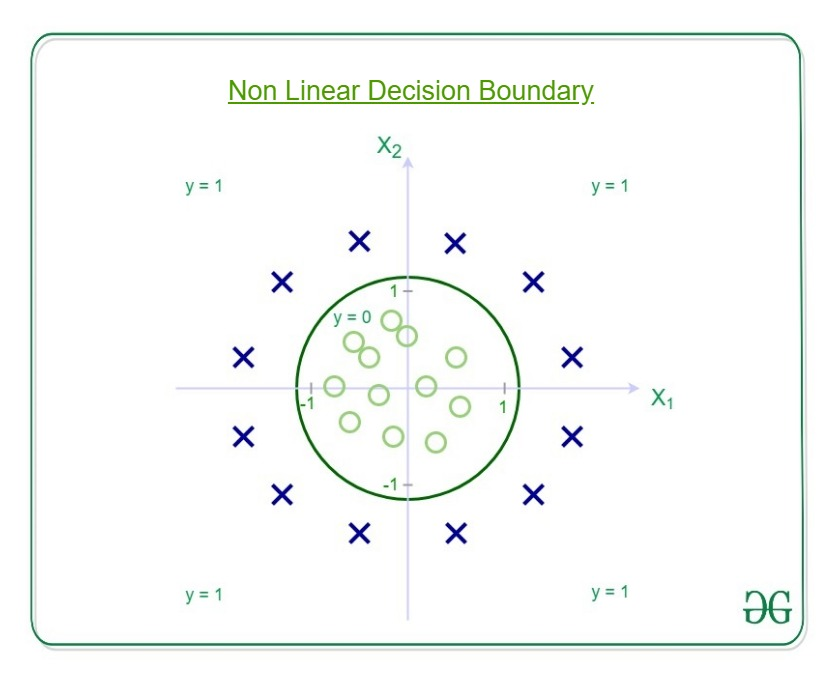Open In App

# ML | Why Logistic Regression in Classification ?

Using Linear Regression, all predictions >= 0.5 can be considered as 1 and rest all < 0.5 can be considered as 0. But then the question arises why classification can’t be performed using it? Problem – Suppose we are classifying a mail as spam or not spam and our output is y, it can be 0(spam) or 1(not spam). In case of Linear Regression, hθ(x) can be > 1 or < 0. Although our prediction should be in between 0 and 1, the model will predict value out of the range i.e. maybe > 1 or < 0. So, that’s why for a Classification task, Logistic/Sigmoid Regression plays its role.

Logistic regression is a statistical method commonly used in machine learning for binary classification problems, where the goal is to predict one of two possible outcomes, such as true/false or yes/no. Here are some reasons why logistic regression is widely used in classification tasks:

Simple and interpretable: Logistic regression is a relatively simple algorithm that is easy to understand and interpret. It can provide insights into the relationship between the independent variables and the probability of a particular outcome.

Linear decision boundary: Logistic regression can be used to model linear decision boundaries, which makes it useful for separating data points that belong to different classes.

Efficient training: Logistic regression can be trained quickly, even with large datasets, and is less computationally expensive than more complex models like neural networks.

Robust to noise: Logistic regression can handle noise in the input data and is less prone to overfitting compared to other machine learning algorithms.

Works well with small datasets: Logistic regression can perform well even when there is limited data available, making it a useful algorithm when dealing with small datasets.

Overall, logistic regression is a popular and effective method for binary classification problems. However, it may not be suitable for more complex classification problems where there are multiple classes or nonlinear relationships between the input variables and the outcome.Here, we plug θTx into logistic function where θ are the weights/parameters and x is the input and hθ(x) is the hypothesis function. g() is the sigmoid function.It means that y = 1 probability when x is parameterized to θ To get the discrete values 0 or 1 for classification, discrete boundaries are defined. The hypothesis function cab be translated asDecision Boundary is the line that distinguishes the area where y=0 and where y=1. These decision boundaries result from the hypothesis function under consideration. Understanding Decision Boundary with an example – Let our hypothesis function beThen the decision boundary looks likeLet out weights or parameters be –So, it predicts y = 1 ifAnd that is the equation of a circle with radius = 1 and origin as the center. This is the Decision Boundary for our defined hypothesis.